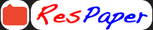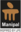Trending ▼   ResFinder# ICSE Class 8 Sample / Model Paper 2021 : Mathematics

2 pages, 14 questions, 0 questions with responses, 0 total responses,00Soumya Roy Sikkim Manipal University (SMU), Gangtok Master of Computer Application Computer Application
+Fave Message
 Home > coolsroy_mdp >Formatting page ...

MADHUSTHALI VIDYAPEETH F.M 40 Time Date : PROMOTIONAL EXAMINATION : 2020-2021 SUBJECT: MATHEMATICS CLASS- VIII Attempt all questions. Each question carries 4 marks Don t give too much time on any question. Q.1 A card is drawn at random from a box containing 12 cards numbered 1, 2, 3, .....,12. Find the probability of drawing: (a)a 5 ; (b)an odd number; (c)a prime number; (d)a negative number; Q.2 If y varies directly as x, and y = 30 when x = 5, find: (a) an equation connecting x and y. (b) the value of y when x = 10. (c) the value of x when y = 54. Q.3 A contractor who has a workforce of 624 men, undertook to complete a building in 11 months. How many extra men must he employ to complete the same job in 8 months? Q.4 The digit in the units place of a two-digit number is 4 times the digit in the tens place. If the digits are reversed and the original number is subtracted from the new number, the difference is 54. What is the original number? Q.5 Q.6 Solve the following equation: Q.7 ABCD is a parallelogram, EF // AC, EDA = 28 & CDF = 24 . Calculate: (i) ACD (ii) DAC (iii) ABC 1Formatting page ...